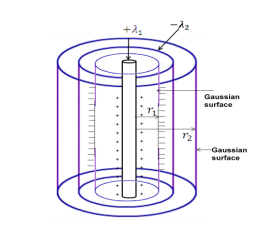A long charged cylinder of linear charge density $+{\lambda }_{1}$ is surrounded by a hollow coaxial conducting cylinder of linear charge density $-{\lambda }_{2}$. Use Gauss's law to obtain expressions for the electric field at a point (i) in the space between the cylinders, and (ii) outside the larger cylinder.                (3) marks

As gauss law states

(1/2) mark(1/2) mark

Difficulty Level:

• 78%
• 19%